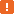# BuiltIn.VarToBool, VarToFloat, VarToInteger, VarToString, VarToWString MethodsThis method is obsolete. See the Remarks section below.

### Description

The `VarToBool`, `VarToFloat`, `VarToInteger`, `VarToString` and `VarToWString` functions are used to convert variant values to a specific type. TestComplete scripts use OLE variant variables which can take different variant types. When they are used as parameters or as terms in an expression, an implicit conversion takes place to assign them the type implied by the context. However, sometimes the script interpreter needs help. For instance, if you mean to concatenate a string-variant with an integer-variant, using the + sign (or & in VBScript), you should convert the second term to string-variant type first:

JavaScript, JScript

resultString = string1 + VarToString(int2);

Python

``resultString = string1 + VarToString(int2)``

VBScript

resultString = string1 & VarToString(int2)

DelphiScript

resultString := string1 + VarToString(int2);

C++Script, C#Script

resultString = string1 + VarToString(int2);

Calling methods, functions or procedures in an Open Application from TestComplete scripts may likewise require preprocessing for their parameters. In most cases, the conversion from the OLE variant to the native application type and back is performed automatically. But there are cases where the script interpreter is unable to determine the needed native type definitely. The two most frequent cases are -

• untyped pointers, for example, `FuncU(varValue)` in Object Pascal or void `FuncU(void * U)` in C++, and
• binary equivalents; for instance parameter declarations A and B below are equivalent:
C++ Object Pascal
`char * A  ` ``` A: PChar```
`char& B  ` `var B : BYTE`

Take the Object Pascal example. A pointer to a char or to a byte are both the addresses of one byte in memory. It just happens that a convention allows us to pass the starting address of an array of chars as a `PChar`. Where application source says `FuncA(A : PChar)`, at runtime nothing indicates if the parameter is a string, or a byte being passed by reference.

Therefore, TestComplete supplies `VarToString`, `VarToWString`, `VarToInteger`, `VarToBool` and ` VarToFloat` so that you may specify the native type of parameters for application routines. For instance, in the following code ` VarToString` specifies that ` FuncA` needs a null-terminated string as a parameter:

JavaScript, JScript

i = "12";
w.MyForm.FuncA(VarToString(i));

Python

``````i = "12"
w.MyForm.FuncA(VarToString(i))``````

VBScript

i = "12"
w.MyForm.FuncA(VarToString(i))

DelphiScript

i := '12';
w.MyForm.FuncA(VarToString(i));

C++Script, C#Script

i = "12";
w["MyForm"]["FuncA"](VarToString(i));

These "functions" are more in the nature of compiler directives. The effect of putting one in a parameter list is to override the interpreter's default conversions. Other ambiguous parameters in the list will also need `VarToxxx`, even though normally they would be converted transparently. For instance,

Delphi

// Declaration
TMyForm = class (...)
procedure FuncA(A : PChar; var C : BYTE);
...
end;

JavaScript, JScript

// Script routine
w.MyForm.FuncA(VarToString("Hello"), myNum); // Error!!!
w.MyForm.FuncA(VarToString("Hello"), VarToInteger(myNum)); // Correct

Python

``````# Script routine
w.MyForm.FuncA(VarToString("Hello"), myNum) # Error!!!
w.MyForm.FuncA(VarToString("Hello"), VarToInteger(myNum)) # Correct``````

VBScript

' Script routine
Call w.MyForm.FuncA(VarToString("Hello"), myNum) ' Error!!!
Call w.MyForm.FuncA(VarToString("Hello"), VarToInteger(myNum)) ' Correct

DelphiScript

// Script routine
w.MyForm.FuncA(VarToString('Hello'), myNum); // Error!!!
w.MyForm.FuncA(VarToString('Hello'), VarToInteger(myNum)); // Correct

C++Script, C#Script

// Script routine
w["MyForm"]["FuncA"](VarToString("Hello"), myNum); // Error!!!
w["MyForm"]["FuncA"](VarToString("Hello"), VarToInteger(myNum)); // Correct

`VarToBool` is typically used in script instructions that compare the value of a property or a field exported from an Open Application. TestComplete reads boolean properties and fields as integers: False is 0, True is any other integer value, e.g. 1 or -2. Without the `VarToBool` conversion, some comparison instructions (typically in VBScript procedures) may produce incorrect results.These methods are obsolete. They are supported for backward compatibility only.

### Declaration

BuiltIn.VarToNNN(Value)

 Value [in] Required Variant Result A value of a specific type.

### Applies To

The method is applied to the following object:

### Parameters

The method has the following parameter:

Value

A variant value to convert.

### Result Value

A value of a specific type.

### Remarks

These methods are obsolete. They are supported for backward compatibility only. To convert variant values to values of specific types, use the methods of the aqConvert object.